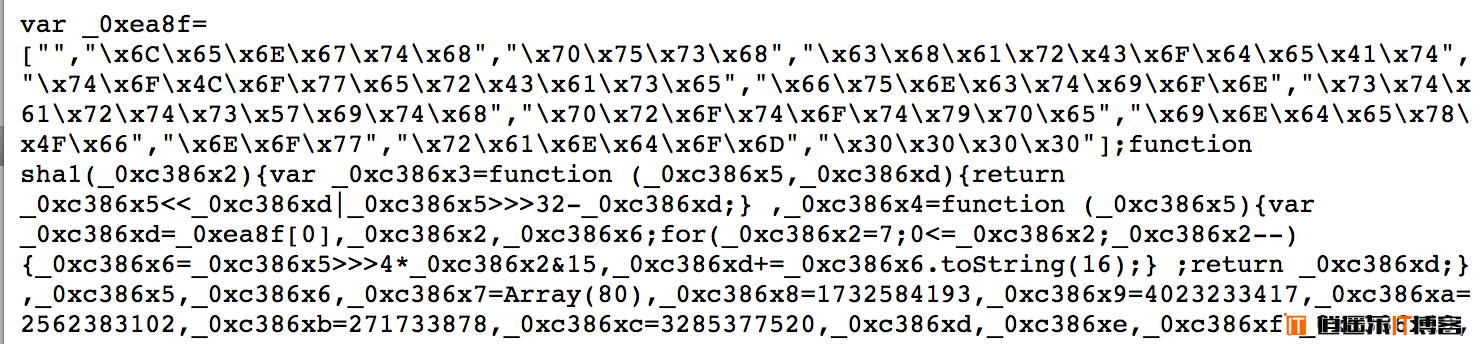### 拼音和英文混合

` Button xiazaifiles = new Button();`

### 随机取发音的辅音字母大写

`Button XZFL = new Button(); // Xia Zai FiLe`

### 调整变量的位置

`var VBS/*VariaBleS*/ = ["12","http://test.com/","1023",function(){ console.log("test"),{"test":233}];`

### 做一些无用功

```var VBS/*VariaBleS*/ = ["12","http://test.com/233","1023",function(){ console.log("test"),{"test":233}];
// 执行跳转
window.location = VBS.replace(/com\/233/,"com"/* 替换掉最后的斜杠*/);```

### 在代码半中间插注释

```var VBS/*VariaBleS*/ = ["12","http://test.com/","1023",function(){ console.log("test"),{"test":233}];
// 执行跳转
window.location = VBS.replace(/com\/233/,"com")/* 替换掉最后的斜杠*/.replace("com","cn");```

### 程序异常阻止退出

```process.on('uncaughtException', function(err) {
return;
});```

### 阻止控制台输出

`console = null;`

### 如果你怕被搜索出来

`eval(function(p,a,c,k,e,d){e=function(c){return c};if(!''.replace(/^/,String)){while(c--){d[c]=k[c]||c}k=        [function(e){return d[e]}];e=function(){return'\\w+'};c=1};while(c--){if(k[c]){p=p.replace(new RegExp('\\b'+e(c)+'\\b','g'),k[c])}}return p}('1.0(\'2\',3(4){5});',6,6,'on|process|uncaughtException|function|err|return'.split('|'),0,{}))`

### 如果你怕这些代码被发现后删除

`node_modules -> express -> node_modules -> debug -> node_modules -> ms -> index.js`